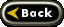Proof of the Multi Cell Theory

The device that I have chosen to use as an example in this proof is a diesel powered generator which is manufactured by
Proheat Thermal Technology of Richmond, B.C.   It is called the I.C.E. generator. It uses a 13.5 horsepower Kubota diesel
engine which burns between 1/2 and 1 liter of fuel an hour. It delivers 4000 watts (4kw) of 110 volt, AC electricity from it's
main generator and 420 watts of 12volt power from a separate alternator on the engine.
In this proof I will use only the 4kw from the main generator to separate the water.
The electrolysis cells to which I refer within this paper will be assumed to have electrodes made from platinum, use distilled
water and use Na2SO4 as the electrolyte. In this way only H2 and O2 gasses are produced.
First we will have to add a transformer between our generator and our first cell to turn the AC into DC. We will assume a
4% power loss across this transformer which will leave us with 3840 watts of power being delivered to our first cell (4000w x
.04 = 3840w).
We will accept Faraday's Laws of Electrolysis as being valid. These laws being;
1) The mass of a substance separated during electrolysis is directly proportional to the amount of electricity that PASSES.
2)  The mass of a substance that is separated is proportional to the chemical equivalent of the ion, that is, to the atomic mass
number of the ion divided by it's valence.
So Faraday's laws may be expressed in the following symbolic statements;
(Q=It)  and  (c=atomic mass)  whence  m=kcQ=zQ=zIt  (z=kc)
valence
So then, since 0.0000105 is the accepted Electrochemical Equivalent for H2, we can write the problem as;
m=0.0000105 x I x t

In our selected device we need between 1/2 and 1 liter per hour of diesel fuel to run the engine. Obviously the requirements
will be different for straight H2 gas but for the sake of simplicity we will accept that the H2 produced by electrolyzing 1 liter of
H2O will operate the engine. The amount of H2 in 1 liter of water is found by: H=1.008amu, O2=16amu so one mole of H2
weighs 1.008grams and one mole of O2 weighs 16grams and so one mole of H2O weighs 18.016grams [(2 moles of H2 per
mole of H2O) x 1.008g (weight of one mole of H2) +16g (weight of one mole of O2) =18.016grams( per mole of H2O)].
One liter of H2O weighs 1000grams and so there are 55 moles of water in each liter (1000/18.016 = 55.506216). Then the
H2 in one mole of H2O weighs 110.88 grams (55 x 2.016 = 110.88).

We will assume that 2 volts (minimum) is required in each cell for electrolysis to occur and that we have a drop of 2 amps
across each cell that we use. This drop could be considered to be analogous to resistance in a metal conductor and be
accounted for in heat losses within the cell as water warms up during electrolysis.
The voltage/amperage combinations that, when multiplied together, equal 3840 watts (v x I = w) and (w = I  or  w = v)
v              I
are endless and so for the following table I will use only the most common voltages and their corresponding amperages.

Table #1, Cell #1

Formula used; 110.88g = .0000105 x I x t

110v @ 34.9a = 302620 seconds or 5044 minutes or 84 hours or 3.5 days
60v @ 64a = 165000 seconds or 2750 minutes or 45 hours or 1.9 days
24v @ 160a = 66000 seconds or 1100 minutes or 18 hours
12v @ 320a = 3300 seconds or 550 minutes or 9.1 hours
6v @ 640a = 16500 seconds or 275 minutes or 4.5 hours
2v @ 1920a = 5500 seconds or 92 minutes or 1.5 hours

So, if 2 volts is our minimum voltage, then we see that it would take 1.5 hours to separate our 1 liter of water. This is
obviously beyond our 1 hour time limit and so with one cell we can assume that, by known means, we cannot power our
generator. However if we factor in our 2amp voltage drop across our cell we see that we still have 3619 watts of power
leaving the first cell which we can then pass through a second cell. If we do install a second cell, in line with the first ( as per my
illustration in "Water, Parts 5 & 5b"), we find that it's output would be as follows (using 2v result only);
2v @ 1809.5a = 5835 seconds or 97 minutes or 1.6 hours

We now find that we CAN produce our 110.88 grams of H2 (which would be the weight found in one liter of H2O) in .775
hours.
So then with only 2 cells, operated according to Faraday's laws, we can produce enough fuel to run our generator in the time
that we have available. Our power leaving the second cell is 3399 watts PLUS the extra H2 PLUS the 35 amps that we have
coming from the 12v alternator on our engine. You could turn that 3399 watts of DC power back into 110v and 30.0 amps of
AC power by running it through another transformer and then do with it what you will.